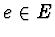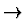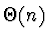CSE 5311 Fall 1999

Quiz #10

1.
(14 points.) Let G = (V, E) be a flow network with source s and sink t in which each edgeis restricted to capacity c(e) = 1. Further suppose that a maximum flow for G has been computed and an edge is now removed from E. Describe how the maximum flow can be efficiently updated and give the run time of your algorithm.

Let the removed edge be (u, v). If (u, v) had no flow, we don't need to do anything. If (u, v) carried flow, the network must be updated. The picture is something like:, where theedge is deleted. There is now one more unit of flow coming into u than is going out (and one more unit of flow going out of v than is coming in). The idea is to try to reroute this unit of flow so that it goes out of u and into v via some other path, if possible. If this is not possible, we must reduce the flow from s to u and from v to t by one unit.

Look for an augmenting path from u to v (not from s to t as we do at every iteration of the basic FordFulkerson method). If there is such a path, augment the flow along that path. If there is no such path, reduce the flow from s to u by augmenting the flow from u to s. That is, find an augmenting path from u to s and augment the flow along that path. (There is definitely such a path, because there is flow from s to u.) Augment the flow from t to v similarly.

The run time is again O(V + E) = O(E) using depth-first search or breadth-first search to find paths from u to s and from t to v.

2.
(6 points.) Analyze the run time of the parallel code below. Also compute the speedup, assuming that the serial run time isand the number of processors p is >= n. In this algorithm assume that there are three arrays (A[1n], B[1n], and C[1k]) used, and each element i of array C is predefined to contain the number of elements from array A equal to i. Each element of arrays B and C are stored on a separate processor.

   ParallelAlg(A, B, C, k)
for each processor j, in parallel
C[j] = C[j] + C[j-1]
for each processor j, in parallel
B[C[A[j]]] = A[j]
C[A[j]] = C[A[j]] - 1


Is this parallel algorithm work efficient? Which sorting technique does this most closely resemble?

The run time is O(1) and the speedup is n. As long as k = n, the parallel algorithm is work efficient and closely resembles Counting Sort.